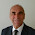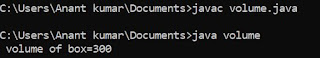## Sunday, 15 April 2018

### Program To Input Three Number From User And Check Which Is Maximum Number In C"

This is program to find maximum number between three number in C in which first of all declare three variable at the starting of the program and then input three number from the user and after that we have write conditions for checking the maximum of three variable.

/* maximum of 3 number*/
# include <stdio.h>
void main ()
{
int a ,b ,c;
clrscr ();
printf ("/n Enter value of a:");
scanf ("%d", & a);
printf ("/n Enter value of b:");
scanf ("%d", & b);
printf ("/n Enter value of c:");
scanf ("%d", & C);
if ( (a>b) && (a>c) )
printf ("/n a is maximum number:");
if ( (b>a) && (b>c) )
printf ("/n b is maximum number:");
if ( (c>a) && (c>b) )
printf ("/n c is maximum number:");
getch();
}

Here we use condition for checking that input number is maximum or not but we can also made this program with the help of ' if else ' statement in using with two condition.

#### 1 comment:

1.A very useful site, which I will bear in mind for future reference, Alan

## Featured post

### Program to find Volume of given box in java

class volume { public static void main (String args []) { int l=5,b=6,h=10; int volume; volume=l*b*h; System.out.println (" vol...